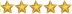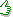At a glanceAuthor: Konrad Hejwosz   How to become an author?Items: 467Rating:Last updated: Dec 30, 2000DescriptionThe name trigonometry has its origin in Greek words trigonon, meaning triangle, and metron, meaning a measure. As it is can be partly deduced from the origin of the name, trigonometry is the branch of mathematics that deals with the relations between the sides and angles of triangles. It is also concerned with solving triangles by means of trigonometric functions. Although the name trigonometry first came into use at the end of the 15th century, its earliest rudiments can be found in records from Egypt and Mesopotamia. Throughout ages, trigonometry has found numerous applications, e.g. a tool for astronomical calculations, surveying lands, measureing distances across rivers, computing mountain heights, etc.Trig Functions together with SuperMemo will help you learn and keep in your memory trignometric ratios, properties and values of the most frequently required angles, reduction formulae, addition formulae, double-angle formulae, tangent of half-angle formulae, product formulae, sums and differences, derivatieves, and integrals of trigonometric functions.With nearly 500 items, the collection serves as a strong basis for those who would like to study the subject in-depth.ExamplesQ: geom: the trig function equal in a right-angled triangle to the ratio of the hypotenuse to the side adjacent an acute angle A: secant Q: geom: Is tangent either even or odd function? A: odd Q: geom: What is the name of the rule of the form: a/sin A = b/sin B = c/sin C = 2R (where: A, B, C - the angles of the triangle; a, b, c - the lengths of the sides opposite them; R - the radius of the circumcircle (about the triangle))? A: sine rule/law of sines Q: T/F: Sine takes negative values in the 2nd quadrant. (math) A: False Q: geom: (cos A)' = [...] A: -sin ACompatibilitySM6 SM7 SM8 SM98 SM99 SM2000 SM2002 Palm SM SMCEtrigfns\$6.00With SuperMemo you can forget about forgetting!